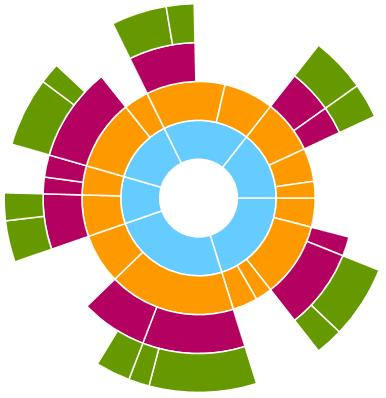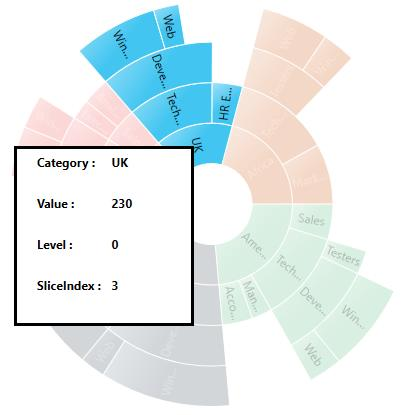# Events in UWP Sunburst Chart (SfSunburstChart)

## SegmentCreated Event

This event occurs when segment is created. You can get the segment details as argument from the `SunburstSegmentCreatedEventArgs` handler.

The following example shows how to set the color for each level after creating the segment.

• c#
• ``````private void chart_SegmentCreated(object sender, SunburstSegmentCreatedEventArgs e)

{

byte r = 180, g = 0, b = 96;
byte r1 = 255, g1 = 153, b1 = 0;
byte r2 = 102, g2 = 153, b2 = 0;
byte r3 = 102, g3 = 204, b3 = 255;
if (e.Segment.CurrentLevel == 0)
e.Segment.Interior = new SolidColorBrush(Color.FromRgb(r3,g3,b3));

else if (e.Segment.CurrentLevel == 1)
e.Segment.Interior = new SolidColorBrush(Color.FromRgb(r1, g1, b1));

else if (e.Segment.CurrentLevel == 2)
e.Segment.Interior = new SolidColorBrush(Color.FromRgb(r, g, b));

else if (e.Segment.CurrentLevel == 3)
e.Segment.Interior = new SolidColorBrush(Color.FromRgb(r2, g2, b2));

}``````## SelectionChanged

This event occurs whenever you select the segment by enabling selection behavior. You can get the selected segment details as argument from `SunburstSelectionChangedEventArgs` handler.

The following examples shows how to set the selected segment information.

``````<sunburst:SfSunburstChart.Behaviors>

<sunburst:SunburstSelectionBehavior EnableSelection="True"
SelectionCursor="Hand"/>
</sunburst:SfSunburstChart.Behaviors>

<Popup x:Name="popup" Placement="MousePoint" Height="180" Width="180" >

<Border Background="White" BorderBrush="Black" BorderThickness="3" >

<Grid Margin="20,5,5,5">

<Grid.RowDefinitions>
<RowDefinition/>
<RowDefinition/>
<RowDefinition/>
<RowDefinition/>
</Grid.RowDefinitions>

<Grid.ColumnDefinitions>
<ColumnDefinition/>
<ColumnDefinition/>
</Grid.ColumnDefinitions>

<TextBlock Text="Category : " FontWeight="Bold" Grid.Row="0" Grid.Column="0"/>
<TextBlock x:Name="category"   FontWeight="Bold" Grid.Row="0" Grid.Column="1"/>
<TextBlock Text="Value : " FontWeight="Bold" Grid.Row="1" Grid.Column="0"/>
<TextBlock  x:Name="value" FontWeight="Bold" Grid.Row="1" Grid.Column="1"/>
<TextBlock Text="Level : " FontWeight="Bold" Grid.Row="2" Grid.Column="0"/>
<TextBlock x:Name="level" FontWeight="Bold" Grid.Row="2" Grid.Column="1"/>
<TextBlock Text="SliceIndex : " FontWeight="Bold" Grid.Row="3" Grid.Column="0"/>
<TextBlock x:Name="sliceIndex" FontWeight="Bold" Grid.Row="3" Grid.Column="1"/>

</Grid>

</Border>

</Popup>``````
``````private void chart_SelectionChanged(object sender, SunburstSelectionChangedEventArgs e)

{

popup.IsOpen = true;
category.Text = e.SelectedSegment.Category.ToString();
value.Text = e.SelectedSegment.Value.ToString();
level.Text = e.SelectedSegment.CurrentLevel.ToString();
sliceIndex.Text = e.SelectedSegment.SliceIndex.ToString();
}``````